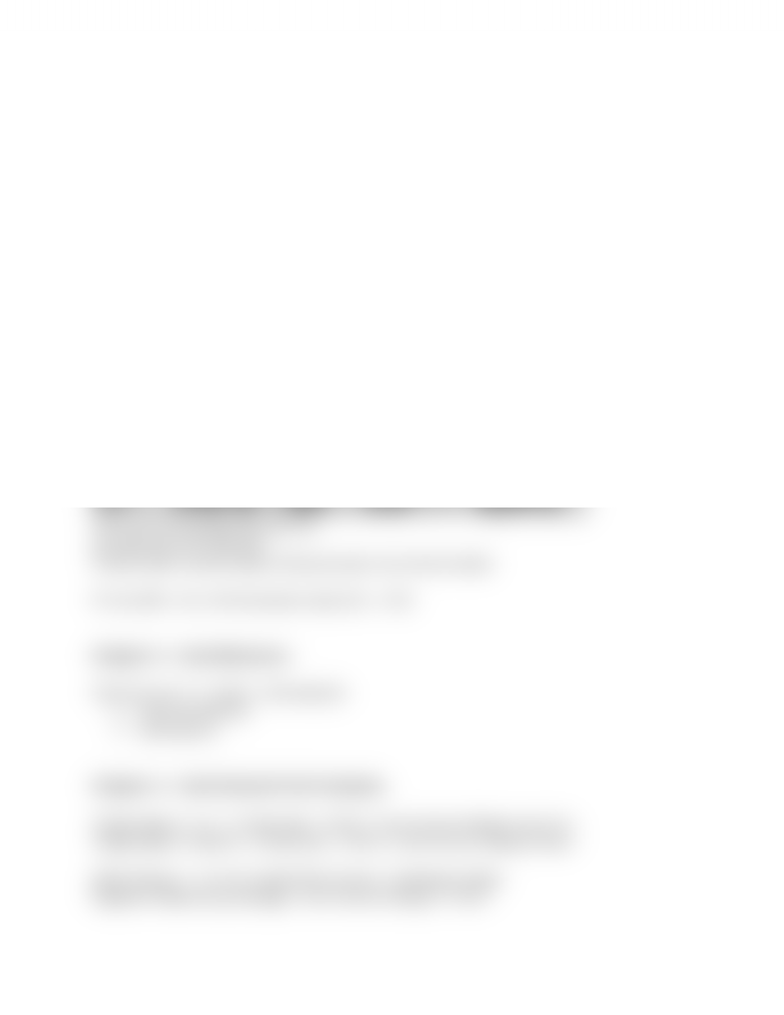Study Guides (400,000)
CA (160,000)
Ryerson (10,000)
ACC 406 (80)

# Study Guide

Department
Accounting
Course Code
ACC 406
Professor
Alison Beavis

This preview shows half of the first page. to view the full 2 pages of the document.Chapter 1 - Introduction
2 - Cost concepts and classifications
3 inventory accounts (Finished, WIP, Raw materials)
3 Cost components (DL, DM, OH)
7 manufacturing Accounting equations
Manufacturing cost = DL + DM + OH (Variable, Fixed)
Revenues
Less: COGS
= GM
Less: Operating expenses
= income
Contribution format Income Statement: (Variable Overhead)
Revenues
Less: Variable costs
= Contribution margin
Less: Fixed costs
= income
Actual VS Estimated cost (Budgeted  Standard [10,11] Predetermined )
Total cost VS Average cost per unit
Variable cost VS fixed cost
Product cost (inventoriable) VS period cost (noninventoriable)
Prime (DM + DL) VS Conversion costs (DL + OH)
Chapter 3 Cost Behaviour
Cost formula: Y = Fixed + (Variable)(X)
High-low Method
Less Square
Chapter 4 Cost-Volume-Profit Analysis
Target sales in unit = (Fixed Cost + Profit) / Contribution Margin per Unit
Target sales in dollars = (Fixed Cost + Profit) / Contribution Margin Ratio
Safety Margin = Current sales Performance Breakeven Sales
Degree of Operating Leverage = Contribution Margin / Profit
www.notesolution.com